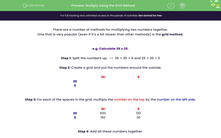# Multiply Using the Grid Method

In this worksheet, students will practise multiplication, with two numbers of up to three-digits, using the grid method.Key stage:  KS 4

Year:  GCSE

GCSE Subjects:   Maths

GCSE Boards:   Pearson Edexcel, OCR, Eduqas, AQA,

Curriculum topic:   Number, Number Operations and Integers

Curriculum subtopic:   Structure and Calculation Calculations with Integers

Difficulty level:#### Worksheet Overview

There are a number of methods for multiplying two numbers together.

One that is very popular (even if it's a bit slower than other methods) is the grid method.

e.g. Calculate 36 x 25.

Step 1: Split the numbers up.  ->  36 = 30 + 6 and 25 = 20 + 5

Step 2: Create a grid and put the numbers around the outside.

 30 6 20 5

Step 3: For each of the spaces in the grid, multiply the number on the top by the number on the left side.

 30 6 20 600 120 5 150 30

Step 4: Add all these numbers together.

 600 +   120 +   150 +     30 900

Before you start this activity, make sure you have a pen and paper handy so that you can create your own grids as we go.

Let's get started!

### What is EdPlace?

We're your National Curriculum aligned online education content provider helping each child succeed in English, maths and science from year 1 to GCSE. With an EdPlace account you’ll be able to track and measure progress, helping each child achieve their best. We build confidence and attainment by personalising each child’s learning at a level that suits them.

Get started